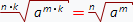## Power formulas:

### 1. Power definition:### 2. Number in zero power:

a0 = 1 при условии, что a ≠ 0

a1 = a

### 4. Negative power transformation: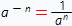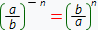### 5. Multiplication in power: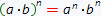if the conditions that n - is integer or a and b are real numbers and even one of them is greater than zero are hold. By using the mathematical notation this statement can be written out like this:an · am = an + m

### 7. Power in power: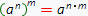### 8. Division in power: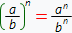### 9. Division with the same bases: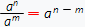### 10. Relationship between powers and logarithms: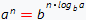### 1. Relationship between powers and radicals (roots):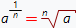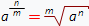### 2. Negative power: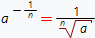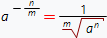### 3. Root from root: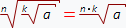### 4. Root from multiplication: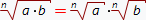### 5. Mutual reducement of the root and power exponents: